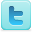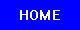﻿ Lenz's Law Experiments for Lesson Plans & Science Fair Projects
Lenz's Law
K-12 Experiments & Background Information
For Science Labs, Lesson Plans, Class Activities, Homework Help & Science Fair Projects
For Elementary, Middle and High School Students and Teachers

Lenz's Law Experiments

Lenz's Law Background Information

## Definition

Lenz's law states that any induced electromotive force will be in the direction such that the flux it creates will oppose the change in the flux that produced it.

## Basics

Lenz's law gives the direction of the induced electromotive force (emf) and current resulting from electromagnetic induction. Heinrich Lenz formulated it in 1834.

Lenz's Law is one consequence of the principle of conservation of energy. To see why, move a permanent magnet towards the face of a closed loop of wire (eg. a coil or solenoid). An electric current is induced in the wire, because the electrons within it are subjected to an increasing magnetic field as the magnet approaches. This produces an emf (Electromotive Force) that acts upon them. The direction of the induced current depends on whether the north or south pole of the magnet is approaching: an approaching north pole will produce an anti-clockwise current (from the perspective of the magnet), and south pole approaching the coil will produce a clockwise current.

To understand the implications for conservation of energy, suppose that the induced currents' directions were opposite to those just described. Then the north pole of an approaching magnet would induce a south pole in the near face of the loop. The attractive force between these poles would accelerate the magnet's approach. This would make the magnetic field increase more quickly, which in turn would increase the loop's current, strengthening the magnetic field, increasing the attraction and acceleration, and so on. Both the kinetic energy of the magnet and the rate of energy dissipation in the loop (due to Joule heating) would increase. A small energy input would produce a large energy output, violating the law of conservation of energy.

This scenario is only one example of electromagnetic induction. Lenz's Law states that the magnetic field of any induced current opposes the change that induces it.

For a rigorous mathematical treatment, see electromagnetic induction and Maxwell's equations.

A demonstration that even a small child can try:

- Find a small electric motor.
- Spin its shaft.
- Connect its wires together (with a paper clip or alligator clip), and spin the shaft again.
- This time, the motor resists turning, because current can flow through its wires.

Source: Wikipedia (All text is available under the terms of the GNU Free Documentation License and Creative Commons Attribution-ShareAlike License.)

Science Fair Projects Resources [Resource]
Electrical Safety [Resource] [Resource]
Electricity Science Fair Projects Books